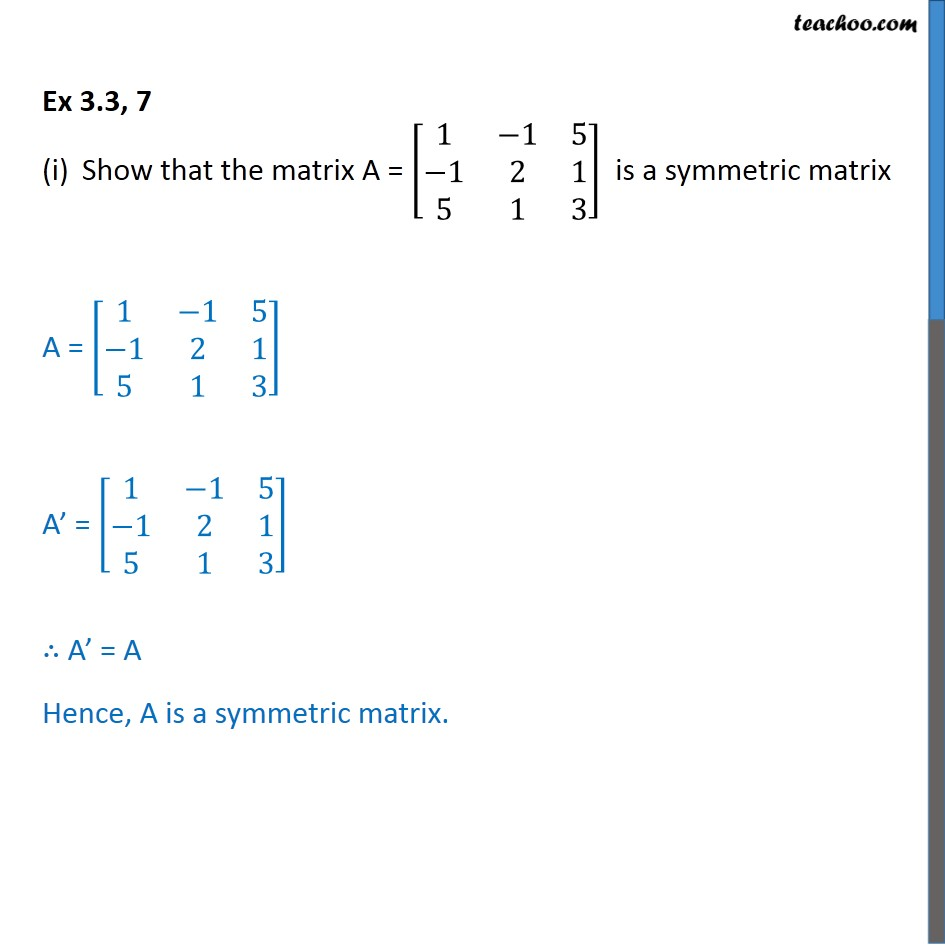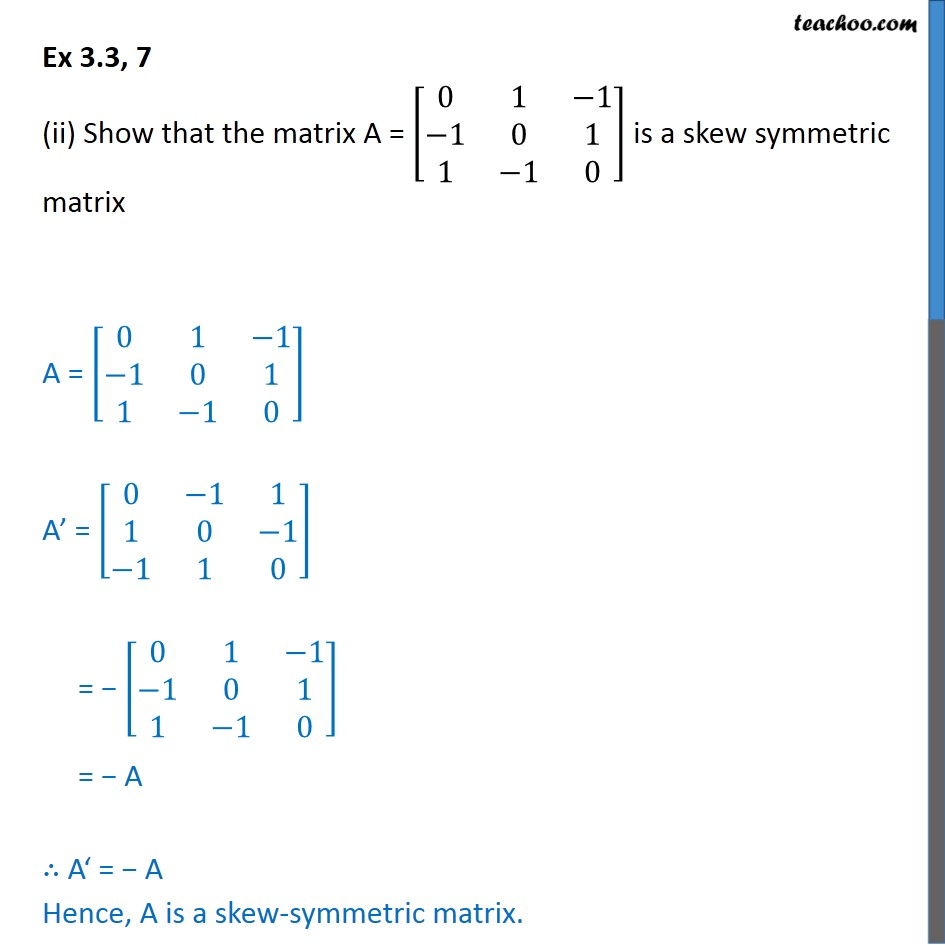1. Chapter 3 Class 12 Matrices
2. Serial order wise
3. Ex 3.3

Transcript

Ex 3.3, 7 Show that the matrix A = [■8(1&−1&5@−1&2&1@5&1&3)] is a symmetric matrix A = [■8(1&−1&5@−1&2&1@5&1&3)] A’ = [■8(1&−1&5@−1&2&1@5&1&3)] ∴ A’ = A Hence, A is a symmetric matrix. Ex 3.3, 7 (ii) Show that the matrix A = [■8(0&1&−1@−1&0&1@1&−1&0)] is a skew symmetric matrix A = [■8(0&1&−1@−1&0&1@1&−1&0)] A’ = [■8(0&−1&1@1&0&−1@−1&1&0)] = − [■8(0&1&−1@−1&0&1@1&−1&0)] = − A ∴ A‘ = − A Hence, A is a skew-symmetric matrix.

Ex 3.3

Chapter 3 Class 12 Matrices
Serial order wise